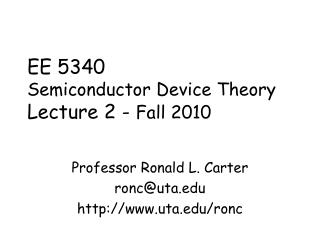Download PresentationEE 5340 Semiconductor Device Theory Lecture 2 - Fall 2010

# EE 5340 Semiconductor Device Theory Lecture 2 - Fall 2010 - PowerPoint PPT PresentationDownload Presentation## EE 5340 Semiconductor Device Theory Lecture 2 - Fall 2010

- - - - - - - - - - - - - - - - - - - - - - - - - - - E N D - - - - - - - - - - - - - - - - - - - - - - - - - - -
##### Presentation Transcript

1. EE 5340Semiconductor Device TheoryLecture 2 - Fall 2010 Professor Ronald L. Carter ronc@uta.edu http://www.uta.edu/ronc

2. Quantum Concepts • Bohr Atom • Light Quanta (particle-like waves) • Wave-like properties of particles • Wave-Particle Duality

3. Wave-particle duality • Davisson and Germer demonstrated wave-like interference phenomena for electrons to complete the duality model

4. Newtonian Mechanics • Kinetic energy, KE = mv2/2 = p2/2m Conservation of Energy Theorem • Momentum, p = mv Conservation of Momentum Thm • Newton’s second Law F = ma = m dv/dt = m d2x/dt2

5. Quantum Mechanics • Schrodinger’s wave equation developed to maintain consistence with wave-particle duality and other “quantum” effects • Position, mass, etc. of a particle replaced by a “wave function”, Y(x,t) • Prob. density = |Y(x,t)• Y*(x,t)|

6. Schrodinger Equation • Separation of variables gives Y(x,t) = y(x)• f(t) • The time-independent part of the Schrodinger equation for a single particle with Total E = E and PE = V. The Kinetic Energy, KE = E - V

7. Solutions for the Schrodinger Equation • Solutions of the form of y(x) = A exp(jKx) + B exp (-jKx) K = [8p2m(E-V)/h2]1/2 • Subj. to boundary conds. and norm. y(x) is finite, single-valued, conts. dy(x)/dx is finite, s-v, and conts.

8. Infinite Potential Well • V = 0, 0 < x < a • V --> inf. for x < 0 and x > a • Assume E is finite, so y(x) = 0 outside of well

9. Step Potential • V = 0, x < 0 (region 1) • V = Vo, x > 0 (region 2) • Region 1 has free particle solutions • Region 2 has free particle soln. for E > Vo , and evanescent solutions for E < Vo • A reflection coefficient can be def.

10. Finite Potential Barrier • Region 1: x < 0, V = 0 • Region 1: 0 < x < a, V = Vo • Region 3: x > a, V = 0 • Regions 1 and 3 are free particle solutions • Region 2 is evanescent for E < Vo • Reflection and Transmission coeffs. For all E

11. Kronig-Penney Model A simple one-dimensional model of a crystalline solid • V = 0, 0 < x < a, the ionic region • V = Vo, a < x < (a + b) = L, between ions • V(x+nL) = V(x), n = 0, +1, +2, +3, …, representing the symmetry of the assemblage of ions and requiring that y(x+L) = y(x) exp(jkL), Bloch’s Thm

12. K-P Potential Function*

13. K-P Static Wavefunctions • Inside the ions, 0 < x < a y(x) = A exp(jbx) + B exp (-jbx) b = [8p2mE/h]1/2 • Between ions region, a < x < (a + b) = L y(x) = C exp(ax) + D exp (-ax) a = [8p2m(Vo-E)/h2]1/2

14. K-P Impulse Solution • Limiting case of Vo-> inf. and b -> 0, while a2b = 2P/a is finite • In this way a2b2 = 2Pb/a < 1, giving sinh(ab) ~ ab and cosh(ab) ~ 1 • The solution is expressed by P sin(ba)/(ba) + cos(ba) = cos(ka) • Allowed valued of LHS bounded by +1 • k = free electron wave # = 2p/l

15. K-P Solutions*

16. K-P E(k) Relationship*

17. Analogy: a nearly-free electr. model • Solutions can be displaced by ka = 2np • Allowed and forbidden energies • Infinite well approximation by replacing the free electron mass with an “effective” mass (noting E = p2/2m = h2k2/2m) of

18. Generalizationsand Conclusions • The symm. of the crystal struct. gives “allowed” and “forbidden” energies (sim to pass- and stop-band) • The curvature at band-edge (where k = (n+1)p) gives an “effective” mass.

19. Silicon BandStructure** • Indirect Bandgap • Curvature (hence m*) is function of direction and band.  is x-dir,  is cube diagonal • Eg = 1.17-aT2/(T+b) a = 4.73E-4 eV/K b = 636K

20. References • *Fundamentals of Semiconductor Theory and Device Physics, by Shyh Wang, Prentice Hall, 1989. • **Semiconductor Physics & Devices, by Donald A. Neamen, 2nd ed., Irwin, Chicago. • M&K = Device Electronics for Integrated Circuits, 3rd ed., by Richard S. Muller, Theodore I. Kamins, and Mansun Chan, John Wiley and Sons, New York, 2003.